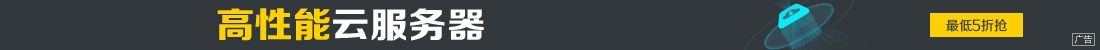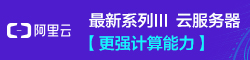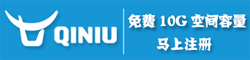# 轻松实现TensorFlow微信跳一跳的AI

••1.需要设备：

Android手机，数据线
Python环境（本例使用3.6.1）
TensorFlow（本例使用1.0.0）

2.大致原理

3.训练过程

4.上代码

```# 输入：100*100的灰度图片，前面的None是batch size，这里都为1
x = tf.placeholder(tf.float32, shape=[None, 100, 100, 1])
# 输出：一个浮点数，就是按压时间，单位s
y_ = tf.placeholder(tf.float32, shape=[None, 1])

# 第一层卷积 12个feature map
W_conv1 = weight_variable([5, 5, 1, 12], 0.1)
b_conv1 = bias_variable(, 0.1)
# 卷积后为96*96*12

h_conv1 = tf.nn.relu(conv2d(x, W_conv1) + b_conv1)
h_pool1 = max_pool_4x4(h_conv1)
# 池化后为24*24*12

# 第二层卷积 24个feature map
W_conv2 = weight_variable([5, 5, 12, 24], 0.1)
b_conv2 = bias_variable(, 0.1)
# 卷积后为20*20*24

h_conv2 = tf.nn.relu(conv2d(h_pool1, W_conv2) + b_conv2)
h_pool2 = max_pool_4x4(h_conv2)
# 池化后为5*5*24

# 全连接层5*5*24 --> 32
W_fc1 = weight_variable([5 * 5 * 24, 32], 0.1)
b_fc1 = bias_variable(, 0.1)
h_pool2_flat = tf.reshape(h_pool2, [-1, 5 * 5 * 24])
h_fc1 = tf.nn.relu(tf.matmul(h_pool2_flat, W_fc1) + b_fc1)

# drapout，play时为1训练时为0.6
keep_prob = tf.placeholder(tf.float32)
h_fc1_drop = tf.nn.dropout(h_fc1, keep_prob)
# 学习率
learn_rate = tf.placeholder(tf.float32)

# 32 --> 1
W_fc2 = weight_variable([32, 1], 0.1)
b_fc2 = bias_variable(, 0.1)
y_fc2 = tf.matmul(h_fc1_drop, W_fc2) + b_fc2

# 因输出直接是时间值，而不是分类概率，所以用平方损失
cross_entropy = tf.reduce_mean(tf.square(y_fc2 - y_))
```

```# 获取屏幕截图并转换为模型的输入
def get_screen_shot():
# 使用PIL处理图片，并转为jpg
im = Image.open(r"./jump_temp.png")
w, h = im.size
# 将图片压缩，并截取中间部分，截取后为100*100
im = im.resize((108, 192), Image.ANTIALIAS)
region = (4, 50, 104, 150)
im = im.crop(region)
# 转换为jpg
bg = Image.new("RGB", im.size, (255, 255, 255))
bg.paste(im, im)
bg.save(r"./jump_temp.jpg")

# 使用TensorFlow转为只有1通道的灰度图
img_data_gray = tf.image.rgb_to_grayscale(img_data)
x_in = np.asarray(img_data_gray.eval(), dtype='float32')

# [0,255]转为[0,1]浮点
for i in range(len(x_in)):
for j in range(len(x_in[i])):
x_in[i][j] /= 255

# 因为输入shape有batch维度，所以还要套一层
return [x_in]
``````while True:

…………

# 每训练100个保存一次
if train_count % 100 == 0:
saver_init.save(sess, "./save/mode.mod")

…………

sess.run(train_step, feed_dict={x: x_in, y_: y_out, keep_prob: 0.6, learn_rate: 0.00005})
```

5.总结

1.样本的按压时间大都分布在300ms到900ms之间，刚开始训练的时候发现不论什么输入，输出都一直很谨慎的停留在600左右，还以为这种方法不可行。不过半个小时后再看发现已经有效果了，对于不同的输入，输出值差距开始变大了。所以…相信卷积网络的威力，多给它点耐心。

2.由于我自己最多玩到100多分，后面的数据没法采集到，所以当后面物体变得越来越小时，这个AI也会变得容易挂掉。理论上说让它自己探索不会有这个瓶颈，只是截屏时间实在难以忍受。

3.目前还是初级的版本，有很多可以优化的地方，比如说识别左上角的分数，如果某次跳跃得分较高，那么可以把这次的学习率增大；检测特殊物体，比如超市音乐盒，就停留几秒再进行下一次跳跃，等等。

https://github.com/zhanyongsheng/LetsJump